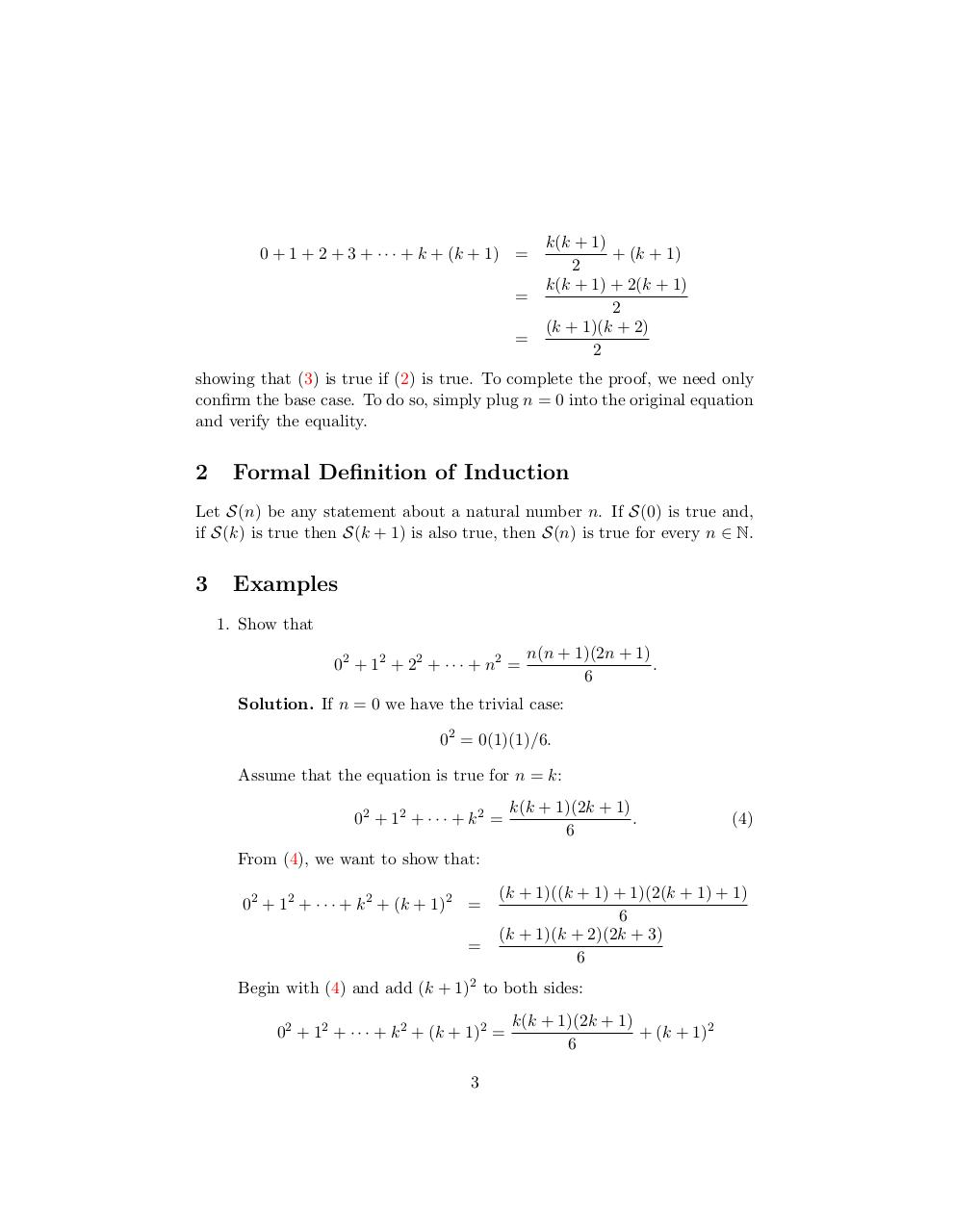# induction.pdfPage 1 2 3 4 5 6 7 8

#### Text preview

0 + 1 + 2 + 3 + · · · + k + (k + 1) =
=
=

k(k + 1)
+ (k + 1)
2
k(k + 1) + 2(k + 1)
2
(k + 1)(k + 2)
2

showing that (3) is true if (2) is true. To complete the proof, we need only
confirm the base case. To do so, simply plug n = 0 into the original equation
and verify the equality.

2

Formal Definition of Induction

Let S(n) be any statement about a natural number n. If S(0) is true and,
if S(k) is true then S(k + 1) is also true, then S(n) is true for every n ∈ N.

3

Examples
1. Show that
02 + 1 2 + 2 2 + · · · + n2 =

n(n + 1)(2n + 1)
.
6

Solution. If n = 0 we have the trivial case:
02 = 0(1)(1)/6.
Assume that the equation is true for n = k:
02 + 12 + · · · + k 2 =

k(k + 1)(2k + 1)
.
6

(4)

From (4), we want to show that:
02 + 12 + · · · + k 2 + (k + 1)2 =
=

(k + 1)((k + 1) + 1)(2(k + 1) + 1)
6
(k + 1)(k + 2)(2k + 3)
6

Begin with (4) and add (k + 1)2 to both sides:
02 + 12 + · · · + k 2 + (k + 1)2 =
3

k(k + 1)(2k + 1)
+ (k + 1)2
6